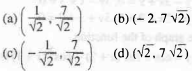The point (4, 1) undergoes the following three transformations successively (I) Reflection about

The point (4, 1) undergoes the following three transformations successively (I) Reflection about the line y = x. (II) Transformation through a distance 2 units along the positive direction of x-axis (III) Rotation through an angle π/4 about the origin in the anticlockwise direction. The final position of the point is given by the co-ordinates.